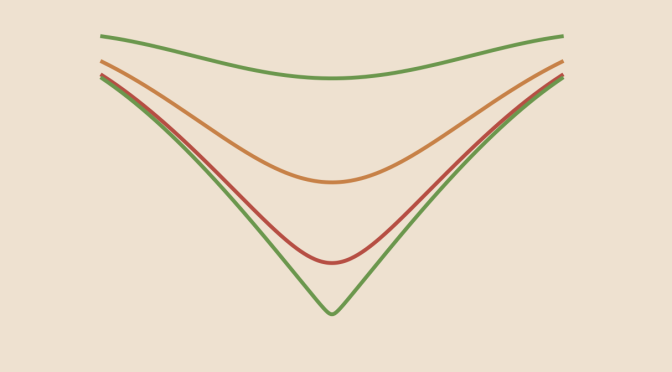Counterexamples around differentiation of sequences of functions

We consider here sequences of real functions defined on a closed interval. Following theorem is the main one regarding the differentiation of the limit.

Theorem: Suppose $$(f_n)$$ is a sequence of functions, differentiable on $$[a,b]$$ and such that $$(f_n(x_0))$$ converges for some point $$x_0 \in [a,b]$$. If $$(f_n^\prime)$$ converges uniformly on $$[a,b]$$, then $$(f_n)$$ converges uniformly on $$[a,b]$$ to a function $$f$$ and for all $$x \in [a,b]$$ $f^\prime(x)=\lim\limits_{n \to \infty} f_n^\prime(x)$ What happens if we drop some hypothesis of the theorem?

If $$(f_n)$$ converges uniformly to $$f$$, and the functions $$f_n$$ are differentiable, does this imply that $$f$$ is also differentiable?

The answer is no. An example is the sequence of functions
$\begin{array}{l|rcl} f_n : & [-1,1] & \longrightarrow & \mathbb{R} \\ & x & \longmapsto & \sqrt{\frac{1}{n^2}+x^2} \end{array}$ These functions are differentiable according to the chain rule as $$g_n(x): x \longmapsto \frac{1}{n^2}+x^2$$ is strictly positive on $$[-1,1]$$. We also have $x^2 \le f_n^2(x) = \frac{1}{n^2}+x^2 \le (\vert x \vert +\frac{1}{n})^2$ hence
$\vert x \vert \le f_n(x) \le \vert x \vert +\frac{1}{n}$ as $$f_n$$ is positive. So by the squeeze test, $$(f_n)$$ converges uniformly to the absolute value function $$f(x) = \vert x \vert$$. But this function is not differentiable at $$0$$. Thus, the uniform limit of differentiable functions need not be differentiable.

If $$(f_n)$$ converges uniformly to $$f$$, and the functions $$f_n$$ are differentiable, does $$(f_n^\prime)$$ also converges?

The answer is again negative as we can see considering the functions $\begin{array}{l|rcl} f_n : & [0,2 \pi] & \longrightarrow & \mathbb{R} \\ & x & \longmapsto & \frac{\sin nx}{\sqrt{n}} \end{array}$ As $$\vert \sin y \vert \le 1$$ for $$y \in \mathbb{R}$$ we get for $$x \in [0,2 \pi]$$
$0 \le \vert f_n(x) \vert \le \frac{1}{\sqrt{n}}$ Hence $$(f_n)$$ converges uniformly to the always vanishing function $$f(x)=0$$. On the other hand, $$f_n^\prime(x)=\sqrt{n} \cos nx$$. And so in particular $$f_n^\prime(0)=\sqrt{n}$$ does not converge. Consequently, $$(f_n^\prime)$$ does not converge pointwise (nor uniformly).

And if $$(f_n^\prime)$$ converges, is the limit $$f^\prime$$?

Again not. Consider $\begin{array}{l|rcl} f_n : & [-1,1] & \longrightarrow & \mathbb{R} \\ & x & \longmapsto & \frac{x}{1+n^2 x^2} \end{array}$ On one hand, we have for all $$x \in [-1,1]$$, $$\vert f_n(x) \vert \le \vert x \vert$$ as $$1+n^2 x^2 > 1$$. Hence $$\vert f_n(x) \vert \le \frac{1}{n}$$ for $$\vert x \vert \le \frac{1}{n}$$.

On the other hand for $$\vert x \vert \ge \frac{1}{n}$$, $$n^2 \vert x \vert \ge n$$ therefore
$\vert f_n(x) \vert \le \frac{1}{n^2 \vert x \vert} \le \frac{1}{n}$ Finally for all $$x \in [-1,1]$$ $\vert f_n(x) \vert \le \frac{1}{n}$ and $$(f_n)$$ converges uniformly to the function $$f(x)=0$$ using the squeeze test.

$$f_n$$ is differentiable with
$f_n^\prime(x) = \frac{1-n x^2}{(1+ n x^2)^2}$ For all $$n \ge 1$$, $$f_n^\prime(0) = 1$$ and for $$x \neq 0$$
$\vert f_n^\prime(x) \vert \le \frac{1+n x^2}{(1+ n x^2)^2} = \frac{1}{1+ n x^2} \underset{n \to \infty}{\to} 0$ Conclusion: $$(f_n)$$ is a sequence of differentiable functions that converges uniformly to the zero function whose sequence of derivatives converges to a function different that the derivative of the limit.